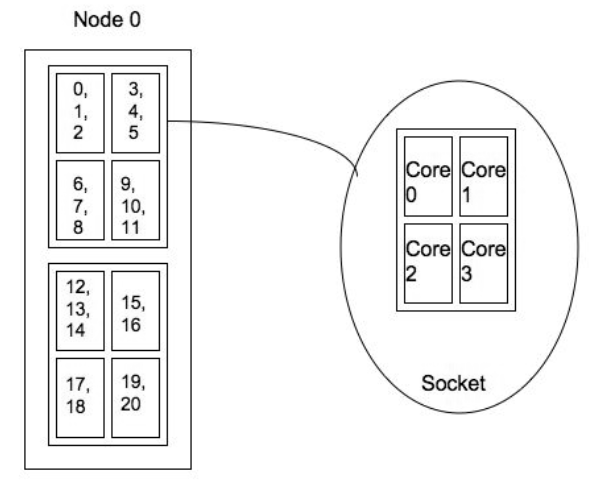# Plane distribution: -m plane=plane_size

The plane distribution allocates tasks in blocks of size plane_size in a round-robin fashion across allocated nodes.

To specify the plane distribution add to the srun command line --distribution=plane=plane_size or -m plane=plane_size where plane_size is the requested plane/block size.

## Examples of plane distributions

In the examples below we assume we have 21 tasks and that the task list is: 0, 1, 2, 3, 4, ..., 19, 20.

On one (1) node: srun -N 1-1 -n 21 -m plane=4 <...>.

Even though the user specified a plane_size of 4 the final plane distribution results in a plane_size of 21, since all the tasks landed on one node.Figure 1: Process layout for srun -N 1-1 -n 21 -m plane=4 <...>

On four (4) nodes: srun -N 4-4 -n 21 -m plane=4 <...>.

The plane distribution with a plane_size of 4 results in the following allocation of the task ids:Figure 2: Process layout for srun -N 4-4 -n 21 -m plane=4 <...>

On four (4) nodes: srun -N 4-4 -n 21 -m plane=2 <...> .

The plane distribution with a plane_size of 2 results in the following allocation of the task ids:Figure 3: Process layout for srun -N 4-4 -n 21 -m plane=2 <...>

## Plane distribution and task affinity

The concept behind this distribution is to divide the clusters into planes. Each plane includes a number of the lowest level of logical processors (CPU, cores, threads depending on the architecture) on each node. We then schedule within each plane first and then across planes.

We ensure that the processes are located correctly by setting the process affinity to the specified/appropriate logical processor. Process affinity is available in Slurm when the task/affinity plug-in is enabled.

On a dual-processor node with quad-core processors (see figure 4) the plane distribution results in:

• One plane if the plane_size=8. In this case the processors are scheduled by first filling up the nodes and then scheduled across the nodes.
• Eight planes if the plane_size=1. In this case we would always schedule across the node first.In a multi-core/hyper-threaded environment, two planes would provide better locality but potentially more contention for other resources.

On the other hand, four planes (scheduling across processors) would minimize contention for cache and memory.

## Examples of plane distributions with process affinity enabled

In the examples below we assume we have 21 tasks and that the task list is: 0, 1, 2, 3, 4, ..., 19, 20.

On one (1) node: srun -N 1-1 -n 21 -m plane=4 --cpu-bind=core <...>. Even though the user specified a plane_size of 4 the final plane distribution results in a plane distribution with plane_size=8.Figure 5: Process layout for srun -N 1-1 -n 21 -m plane=4 --cpu-bind=core <...>.

On four (4) nodes: srun -N 4-4 -n 21 -m plane=4 --cpu-bind=core <...>. The plane distribution with a plane_size of 4 results in the following allocation of the task ids:Figure 6: Process layout for srun -N 4-4 -n 21 -m plane=4 --cpu-bind=core <...>.

On four (4) nodes: srun -N 4-4 -n 21 -m plane=2 --cpu-bind=core <...> . The plane distribution with a plane_size of 2 results in the following allocation of the task ids:Figure 7: Process layout for srun -N 4-4 -n 21 -m plane=2 --cpu-bind=core <...>.